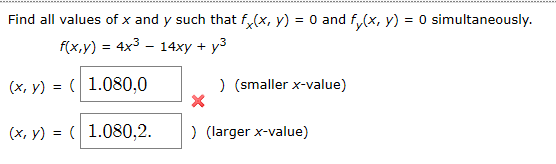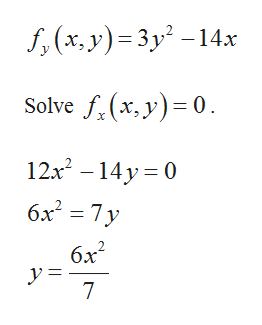# Find all values of x and y such that f(x, y) = 0 and f(x, y) = 0 simultaneously.f(x,y) 4x314xyy3(x, y) = 1.080,0)(smaller x-value)(x, y) = 1.080,2) (larger x-value)

Questionhelp_outlineImage TranscriptioncloseFind all values of x and y such that f(x, y) = 0 and f(x, y) = 0 simultaneously. f(x,y) 4x3 14xyy3 (x, y) = 1.080,0 )(smaller x-value) (x, y) = 1.080,2 ) (larger x-value) fullscreen
check_circleExpert Solution
Step 1

The given function is,

Step 2

Differentiate with respect to x.

Step 3

Differentiate with respe...help_outlineImage Transcriptionclosef, (x. y) 3y2-14x Solve f(x, y) 0 12x2 14y 0 6x2 7 y 6x2 7 fullscreen

### Want to see the full answer?

See Solution

#### Want to see this answer and more?

Solutions are written by subject experts who are available 24/7. Questions are typically answered within 1 hour*

See Solution
*Response times may vary by subject and question
Tagged in

### Calculus